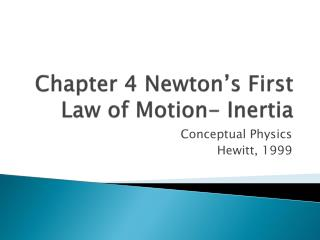# Chapter 4 Newton’s First Law of Motion- Inertia - PowerPoint PPT PresentationDownload PresentationChapter 4 Newton’s First Law of Motion- Inertia

Chapter 4 Newton’s First Law of Motion- InertiaDownload Presentation## Chapter 4 Newton’s First Law of Motion- Inertia

- - - - - - - - - - - - - - - - - - - - - - - - - - - E N D - - - - - - - - - - - - - - - - - - - - - - - - - - -
##### Presentation Transcript

1. Chapter 4 Newton’s First Law of Motion- Inertia Conceptual Physics Hewitt, 1999

2. 4.1 Aristotle on Motion(400B.C.) • Divided motion into two categories: • Natural motion- falling (boulders) or rising (smoke) • Violent motion- applying a force to make something move, “against its nature”

3. 4.2 Copernicus on Motion (1543A.D.) • Heliocentric view- earth around sun • Worked in secret to avoid persecution

4. 4.3 Galileo on Motion(1600’s A.D.) • Force- any push or pull • Friction- force that acts when two things physically interact • Only when friction is present will something have its state of motion changed • Inertia- every object resists change in its state of motion (velocity) • If moving, wants to keep moving • If stationary, wants to sit still

5. Friction Ramp

6. 4.4 Newton’s Law of Inertia • “Every object continues in its state of rest or uniform velocity as long as no net force acts on it.”

7. 4.5 Mass- A Measure of Inertia • Example: Kick an empty paint can and it flies. Kick a paint can filled with paint and you break your toe. • The can with more “stuff” in it has more inertia: more resistance to change in motion (or lack of motion). • Mass is not volume • Mass- measured in kilograms (kg) and is a statement of how much matter it contains • Most fundamental measurement of quantity of matter • Independent of location • Volume- measured in milliliters (mL) or liters (L) and is a statement of how much space the matter takes up

8. Mass is not weight • Weight- the force of gravity on an object • On Earth, Mr. Latham has a mass of 85kg and 185lbs • In deep space, far away from anything, he still has a mass of 85kg, but no weight (weightless) • Weightlessness- your apparent weight is 0N • If your “a”=g • If your “a”≈0m/s2 • Think free falling • http://www.youtube.com/watch?v=2V9h42yspbo

9. Mass is not weight

10. Mass & Force • One kg weighs 9.8 Newtons (on Earth) • 1kg = 9.8N • Force is measured in Newtons (N) • Force of gravity (weight) = (mass)(acceleration due to gravity) • Fg=mg • Remember that on Earth a=g=9.8m/s2 • On the Earth’s moon, g=1.63m/s2

11. 4.6 Net Force • Net force- sum of all of the forces acting on the object • Force (free-body) diagram- shows vectors of all forces involved in motion • Use arrows, length matters, to show the force(s) involved • Helps us visualize what’s going on

12. 4.7 Equilibrium- When Net Force Equals Zero • Normal force- a supporting force: a table, the earth, etc. • Anything that holds an object (or you) up • Typically can support much more than just that one object • We only talk about the force that is used, not what is available • Labeled as FN • Equilibrium- when all of the forces cancel each other out

13. 4.8 Vector Addition of Forces • As the angle gets larger, the resultant force is constant, but the actual forces must get larger as well • Hanging from a vertical string is easier than from a horizontal string

14. Assessments & Formula • F=ma (force) • Fg=mg (weight) • Fnet=F1+F2 • gE=9.8m/s2 • gM=1.63m/s2 • Review Questions 1-20 • Think & Explain 1-12 • Conceptual Development 4-1, 4-2, 4-3